List of Statistical Procedures

ANOVA (Analysis of Variance):

Analysis of variance (ANOVA) is a useful tool which helps the user to identify sources of variability from one or more potential sources, sometimes referred to as "treatments" or "factors".

One-Way ANOVA

The one-way ANOVA is a method of analysis that requires multiple experiments or readings to be taken from a source that can take on two or more different inputs or settings. The one-way ANOVA performs a comparison of the means of a number of replications of experiments performed where a single input factor is varied at different settings or levels. The object of this comparison is to determine the proportion of the variability of the data that is due to the different treatment levels or factors as opposed to variability due to random error. The model deals with specific treatment levels and is involved with testing the null hypothesiswhere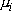represents the level mean. Basically, rejection of the null hypothesis indicates that variation in the output is due to variation between the treatment levels and not due to random error. If the null hypothesis is rejected, there is a difference in the output of the different levels at a significanceand it remains to be determined between which treatment levels the actual differences lie.

Top

Box Plot: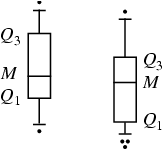A box-and-whisker plot (sometimes called simply a box plot) is a histogram-like method of displaying data, invented by J. Tukey. To create a box-and-whisker plot, draw a box with ends at the quartiles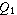and. Draw the statistical median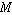as a horizontal line in the box. Now extend the "whiskers" to the farthest points that are not outliers (i.e., that are within 3/2 times the interquartile range ofand). Then, for every point more than 3/2 times the interquartile range from the end of a box, draw a dot. If two dots have the same value, draw them side by side.

Top

Correlation Matrix:

If we have p parameters (variables) each having n data points then the matrix obtained by computing Pearson's Correlation Coefficient for all possible pairs is called the Correlation matrix. This matrix must be symmetric (because correlation between X1 and X2 must be same as the correlation between X2 and X1) and all the diagonal elements must be equal to one. The order of the matrix will be pXp.

Matrix Plot: The combined scatter plot (XY plot) of all the p parameters (variables) is called the matrix plot. An example when p=5 and n=1000 is given below.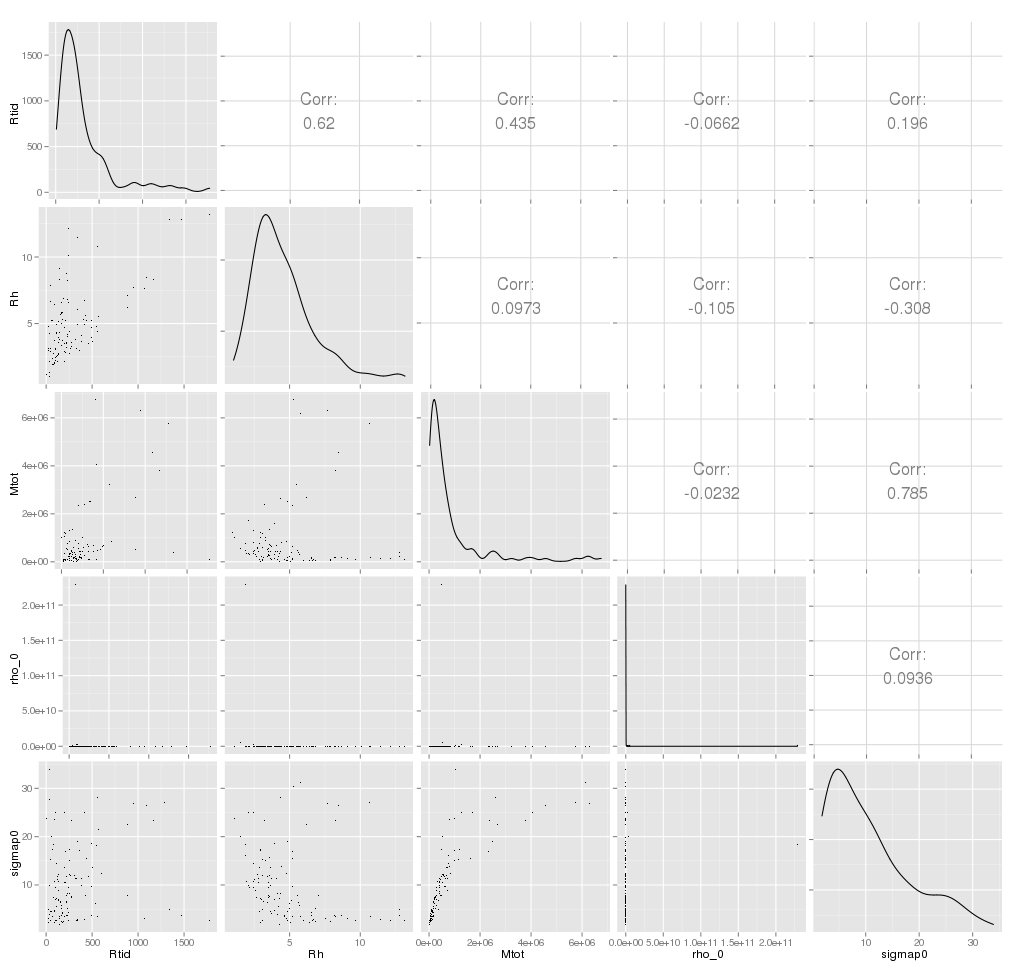Top

Covariance:

Covariance provides a measure of the strength of the correlation between two or more sets of random variates. The covariance for two random variatesand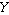, each with sample size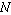, is defined by the expectation value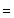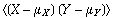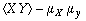= r σx σY

where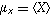and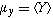are the respective means, which can be written out explicitly as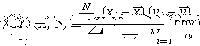For uncorrelated variates,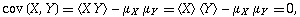so the covariance is zero. However, if the variables are correlated in some way, then their covariance will be nonzero. In fact, if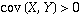, thentends to increase asincreases, and if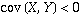, thentends to decrease asincreases. Note that while statistically independent variables are always uncorrelated, the converse is not necessarily true.

Top

Empirical Distribution Function:

In statistics, an empirical distribution function is a cumulative probability distribution function that concentrates probability 1/n at each of the n numbers in a sample.

Let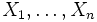be random variables with realizations.The empirical distribution function Fn(x) based on sampleis a step function defined by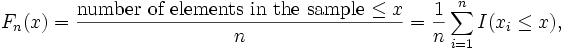where I(A) is an indicator function

Factor Analysis:

Factor Analysis is a dimensionality-reduction technique that aims to find informative combinations of multivariate data using the rotation techniques used in Principal Component Analysis (PCA). While the primary aim to PCA is to find projections of the variables along the direction of maximum variance, Factor Analysis aims to find latent factors that influence the data.
As the solution of Factor Analysis cannot be obtained analytically, the iterative solution depends on number of starting values of factors, the maximum number of iterations permitted to reach the error minima and the number of factors to be extracted from the data. The output consists of loadings for the factors extracted and a hypothesis test tocheck if the number of factors extracted is sufficient.

Top

Hierarchical Clustering:

Given a set of N variables (or objects if N is smalla) to be clustered, and an NxN distance (or similarity) matrix, the basic process of Johnson's (1967) hierarchical clustering is this:

1. Start by assigning each variable to its own cluster, so that if you have N variables, you now have N clusters, each containing just one item. Let the distances (similarities) between the clusters equal the distances (similarities) between the variables they contain.
2. Find the closest (most similar) pair of clusters and merge them into a single cluster, so that now you have one less cluster.
3. Compute distances (similarities) between the new cluster and each of the old clusters.
4. Repeat steps 2 and 3 until all variables are clustered into a single cluster of size N.
5. By looking at the graphical presentation of the distances computed at all the steps (called a dendrogram) and also depending on the physical constraints decide the the step where one should stop. That step will give the optimum number of clusters.

Step 3 can be done in different ways, which is what distinguishes single-link from complete-link and average-link clustering. In single-link clustering (also called the connectedness or minimum method), we consider the distance between one cluster and another cluster to be equal to the shortest distance from any member of one cluster to any member of the other cluster. If the data consist of similarities, we consider the similarity between one cluster and another cluster to be equal to the greatest similarity from any member of one cluster to any member of the other cluster. In complete-link clustering (also called the diameter or maximum method), we consider the distance between one cluster and another cluster to be equal to the longest distance from any member of one cluster to any member of the other cluster. In average-link clustering, we consider the distance between one cluster and another cluster to be equal to the average distance from any member of one cluster to any member of the other cluster.

Top

Histogram:

In statistics, a histogram is a graphical display of tabulated frequencies. A histogram is the graphical version of a table which shows what proportion of cases fall into each of several or many specified categories. The categories are usually specified as non overlapping intervals of some variable. The categories (bars) must be adjacent

Top

Independent Component Analysis:

Independent Component Analysis (ICA) is a dimensionality reduction technique that aims to separate out different signals that constitute a mixed source. A major point differentiating this technique from other dimension reducing techniques is that it extracts statistically independent, non-Gaussian signals. An efficient implementation of this technique, known as FastICA, is used in the analysis here details to which can be found in Help.

Top

K-Means Clustering:

K-means (MacQueen, 1967) is one of the simplest unsupervised learning algorithms that solve the well known clustering problem. The procedure follows a simple and easy way to classify the objects of a given data set through a certain number of clusters (assume k clusters) fixed a priori. The main idea is to define k centroids, one for each cluster. These centroids should be placed in a cunning way because of different location causes different result. So, the better choice is to place them as much as possible far away from each other. The next step is to take each point belonging to a given data set and associate it to the nearest centroid. When no point is pending, the first step is completed and an early groupage is done. At this point we need to re-calculate k new centroids as barycenters of the clusters resulting from the previous step. After we have these k new centroids, a new binding has to be done between the same data set points and the nearest new centroid. A loop has been generated. As a result of this loop we may notice that the k centroids change their location step by step until no more changes are done. In other words centroids do not move any more.
Finally, this algorithm aims at minimizing an objective function, in this case a squared error function. The objective function,

where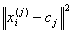is a chosen distance measure between a data point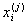and the cluster centre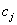, is an indicator of the distance of the n data points from their respective cluster centres. The algorithm is composed of the following steps:

 Place K points into the space represented by the objects that are being clustered. These points represent initial group centroids. Assign each object to the group that has the closest centroid. When all objects have been assigned, recalculate the positions of the K centroids. Repeat Steps 2 and 3 until the centroids no longer move. This produces a separation of the objects into groups from which the metric to be minimized can be calculated.

Although it can be proved that the procedure will always terminate, the k-means algorithm does not necessarily find the most optimal configuration, corresponding to the global objective function minimum. The algorithm is also significantly sensitive to the initial randomly selected cluster centres. The k-means algorithm can be run multiple times to reduce this effect.

Top

Kernel Smoothing

Estimation of functions such as regression functions or probability density functions. Kernel-based methods are most popular non-parametric estimators. It can uncover structural features in the data which a parametric approach might not reveal.

Univariate kernel density estimator:

Given a random sample X1; : : : ;Xn with a continuous, univariate density f, the kernel density estimator is

f(x,h) = (1/(nh)) ∑1n K ((x-Xi)/h)

with kernel K and bandwidth h. Under mild conditions (h must decrease with increasing n) the kernel estimate converges in probability to the true density.

The Kernel K can be a proper pdf. Usually chosen to be unimodal and symmetric

about zero. Center of kernel is placed right over each data point. Influence of each data point is spread about its neighborhood. Contribution from each point is summed to overall estimate.

The band width h is a Scaling factor. It controls how wide the probability mass is spread around a point. It also controls the smoothness or roughness of a density estimate. Bandwidth selection bears danger of under- or oversmoothing.

Kolmogorov Smirnov one sample test:

The test for goodness of fit usually involves examining a random sample from some unknown distribution in order to test the null hypothesis that the unknown distribution function is in fact a known, specified function. We usually use Kolmogorov-Smirnov test to check the normality assumption in Analysis of Variance. However it can be used for other continuous distributions also. A random sample X1,X2, . . . , Xn is drawn from some population and is compared with F*(x) in some way to see if it is reasonable to say that F*(x) is the true distribution function of the random sample.

One logical way of comparing the random sample with F*(x) is by means of the empirical distribution function S(x). Let X1,X2, . . . , Xn be a random sample. The empirical distribution function S(x) is a function of x, which equals the fraction of Xis that are less than or equal to x for each x The empirical distribution function S(x) is useful as an estimator of F(x), the unknown distribution function of the Xis.

We can compare the empirical distribution function S(x) with hypothesized distribution function F*(x) to see if there is good agreement.  One of the simplest measures is the largest distance between the two functions S(x) and F*(x), measured in a vertical direction. This is the statistic suggested by Kolmogorov (1933).

Let the test statistic T be the greatest (denoted by "sup" for supremum) vertical distance between S(x) and F(x).In symbols we say

T = sup x | F*(x) - S(x) |

For testing H0 : F(x) = F*(x) for all x

H1 : F(x) ¹ F*(x) for at least one value of x

If T exceeds the 1-á quantile as given by Table then we reject H0 at the level of significance á. The approximate p-value can be found by interpolation in Table.

Emample:

A random sample of size 10 is obtained: X1 = 0.621,X2 = 0.503,X3 =

0.203,X4 = 0.477,X5 = 0.710,X6 = 0.581,X7 = 0.329,X8 = 0.480,X9 =

0.554,X10 = 0.382.The null hypothesis is that the distribution function

is the uniform distribution function .The mathematical expression for the

hypothesized distribution function is

F*(x) = 0, if x < 0

x, if 0 ≤ x < 1

1, if  1≤ x

Formally , the hypotheses are given by

H0 : F(x) = F(x) for all x from −∞ to ∞

H1 : F(x) /= F(x) for at least one value of x

where F(x) is the unknown distribution function Common to the Xis

and F(x) is given by above equation.

The Kolmogorov test for goodness of fit is used. The critical region of size α = 0.05 corresponds to values of T greater than the 0.95 quantile 0.409, obtained from Table for n=10. The value of T is obtained by graphing the empirical distribution function S(x) on the top of the hypothesized distribution function F*(x).The largest vertical distance is 0.290, which occurs at x = 0.710 because S(0.710) = 1.000 and F*(0.710)=0.710.In other words,

T = sup x | F*(x) − S(x) |

= | F*(0.710) − S(0.710) | = 0.290

Since T=0.290 is less than 0.409, the null hypothesis is accepted. In other words, the unknown distribution F(x) can be considered to be of the form F*(X) on the basis of the given sample. The p value is seen, from Table, to be larger than 0.20.

Top

Kolmogorov Smirnov two sample test:

Perform a Kolmogorov-Smirnov two sample test that two data samples come from the same distribution. Note that we are not specifying what that Common distribution is.

The two sample K-S test is a variation of one sample test. However, instead of comparing an empirical distribution function to a theoretical distribution function, we compare the two empirical distribution functions. That is,

D=sup x | S1(x) − S2(x)|

where S1 and S2 are the empirical distribution functions for the two samples. Note that we compute S1 and S2 at each point in both samples (that is both S1 and S2 are computed at each point in each sample).

The hypothesis regarding the distributional form is rejected if the test statistic, D, is greater than the critical value obtained from a table. There are several variations of these tables in the literature that use somewhat different scaling for the K-S test statistic and critical regions. These alternative formulations should be equivalent, but it is necessary to ensure that the test statistic is calculated in a way that is consistent with how the critical values were tabulated.

Top

Kruskal Wallis two sample test:

The Kruskal-Wallis test is a nonparametric test used to compare three or more samples. It is used to test the null hypothesis that all populations have identical distribution functions against the alternative hypothesis that at least two of the samples differ only with respect to location (median), if at all.

It is the analogue to the F-test used in analysis of variance. While analysis of variance tests depend on the assumption that all populations under comparison are normally distributed, the Kruskal-Wallis test places no such restriction on the comparison.

The Kruskal-Wallis test statistic for k samples, each of size ni is: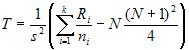- where N is the total number (all ni) and Ri is the sum of the ranks (from all samples pooled) for the ith sample and: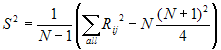The null hypothesis of the test is that all k distribution functions are equal. The alternative hypothesis is that at least one of the populations tends to yield larger values than at least one of the other populations.

Assumptions:

•    random samples from populations
•    independence within each sample
•    mutual independence among samples
•    measurement scale is at least ordinal
•    either k population distribution functions are identical, or else some of the populations tend to yield larger values than other populations

The test statistic for the Kruskal-Wallis test is T. This value is compared to a table of critical values based on the sample size of each group. If T exceeds the critical value  at some significance level (usually 0.05) it means that there is evidence to reject the null hypothesis in favor of the alternative hypothesis.

Mean and Standard deviation:

Mean: The arithmetic mean of a set of values is the quantity Commonly called "the" mean or the average. Given a set of samples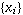, (i=1,2,...N) the arithmetic mean is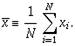When viewed as an estimator for the mean of the underlying distribution (known as the population mean), the arithmetic mean of a sample is called the sample mean.

For a continuous distribution function, the arithmetic mean of the population, denoted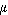, and called the population mean of the distribution, is given by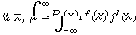Similarly, for a discrete distribution,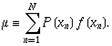The sample meanis an estimate of the population mean μ.

Standard deviation:

In probability and statistics, the standard deviation is a measure of the mean distance of values in a data set from their mean. For example, in the data set (2, 4), the mean is 3 and the standard deviation is 1. Standard deviation is the most Common measure of statistical dispersion, measuring how spread out the values are in a data set. If the data points are all close to the mean, then the standard deviation is low (closer to zero). If many data points are very different from the mean, then the standard deviation is high (further from zero). If all the data values are equal, then the standard deviation will be zero. The standard deviation has no maximum value although it is limited for most data set.

The standard deviation is defined as the square root of the variance. This means it is the root mean square (RMS) deviation from the arithmetic mean. The standard deviation is always a positive number (or zero) and is always measured in the same units as the original data. For example, if the data are distance measurements in meters, the standard deviation will also be measured in meters

The sample standard deviation is given byAnd the population standard deviation is given by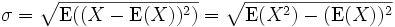where E(X) is the expected value of X.

Median:

The median is the middle of a distribution: half the scores are above the median and half are below the median. The median is less sensitive to extreme scores than the mean and this makes it a better measure than the mean for highly skewed distributions. The median income is usually more informative than the mean income, for example.

When there is an odd number of numbers, the median is simply the middle number. For example, the median of 2, 4, and 7 is 4.

When there is an even number of numbers, the median is the mean of the two middle numbers. Thus, the median of the numbers 2, 4, 7, 12 is (4+7)/2 = 5.5.

Top

One and two sample t-tests:

One Sample( test for one mean value):

Let X1,X2,...Xn be a random sample drawn from a Normal population with mean µ and sd σ. Student's t test is used to compare the unknown mean of the population (µ) to a known number (µ0). So here the Null hypothesis is  Ho:  µ =µagainst the alternative H1: µ is not equal to µ0.

Test statistic (population standard deviation σ is known):

The formula for the Z-test is

Z = √n(Sample mean- µ0) / σ

Z has a Normal distribution with mean 0 and variance 1.

Test statistic (population standard σ deviation is unknown):

The formula for t test is t = √n(Sample mean- µ0) / s

Where s is the sample standard devation.

The statistic t follows t distribution with n-1 degrees of freedom, where n is the number of observations.

Decision of the z or t-test: If the p-value associated with the z or t-test is small (usually set at p < 0.05), there is evidence to reject the null hypothesis in favor of the alternative. In other words, there is evidence that the mean is significantly different than the hypothesized value i.e. the test is significant. If the p-value associated with the z or t-test is not small (p > 0.05), there is not enough evidence to reject the null hypothesis, and you conclude that there is evidence that the mean is not different from the hypothesized value i.e. the test is not significant.

Two sample ( test for equality of two means )

Suppose we have two independent samples The unpaired t method tests the null hypothesis that the population means related to two independent, random samples from two independent approximately normal distributions are equal against the alternative that they are unequal (as in the one sample case).

Assuming equal variances, the test statistic is calculated as: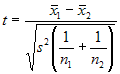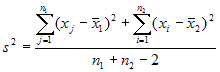where x bar 1 and x bar 2 are the sample means, s² is the pooled sample variance, n1 and n2 are the sample sizes and t follows  Student t distribution with n1 + n2 - 2 degrees of freedom.

Paired Sample (from Bivariate Normal Distribution):

The paired t test provides a hypothesis test of the difference between population means for a pair of random samples whose differences are approximately normally distributed.

The test statistic is calculated as: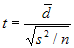where d bar is the mean difference, s² is the sample variance, n is the sample size and t follows a paired t distribution  with n-1 degrees of freedom.

The decision can be taken exactly in a similar way as in the one sample situation.

Optimum 'k' for k-Means:

k-Means clustering is an exploratory tool that helps to discover features in a dataset. Optimum 'k' for k-Means is a tool to help determine the optimum number of clusters that can be extracted from data. This tool works by performing k-Means clustering on the dataset for varying number of clusters (default from 1 to 10) and chooses the optimum number based on the distance between points in a cluster. Inferring directly from this distance can sometimes lead to ambiguity which is why it is transformed using a Distortion Factor. Multiple values of Distortion can (and should) be entered by separating them with a comma.
The output for the test displays the optimum number of clusters both textually and graphically.

Top

Pair Plots:

Scatter plots of the values being compared are generated for each pair of coefficients in x. Different symbols (colors) are used for each object being compared and values corresponding to the same group are joined by a line, to facilitate comparison of fits. If only two coefficients are present then it is equivalent to  xyplot.

Pearson, Kendall and Spearman correlation:

Correlation is a statistical technique which can show whether and how strongly pairs of variables are related. For example, height and weight are related - taller people tend to be heavier than shorter people. The relationship isn't perfect. People of the same height vary in weight, and you can easily think of two people you know where the shorter one is heavier than the taller one. Nonetheless, the average weight of people 5'5'' is less than the average weight of people 5'6'', and their average weight is less than that of people 5'7'', etc. Correlation can tell you just how much of the variation in peoples' weights is related to their heights.

Although this correlation is fairly obvious your data may contain unsuspected correlations. You may also suspect there are correlations, but don't know which are the strongest. An intelligent correlation analysis can lead to a greater understanding of your data..

Like all statistical techniques, correlation is only appropriate for certain kinds of data. Correlation works for data in which numbers are meaningful, usually quantities of some sort. It cannot be used for purely categorical data, such as gender, brands purchased or favorite color.

The correlation coefficient r (also called Pearson's product moment correlation after Karl Pearson) is calculated by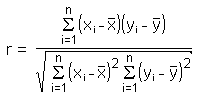The main result of a correlation is called the correlation coefficient (or "r"). It ranges from -1.0 to +1.0. The closer r is to +1 or -1, the more closely the two variables are related.

If r is close to 0, it means there is no relationship between the variables. If r is positive, it means that as one variable gets larger the other gets larger. If r is negative it means that as one gets larger, the other gets smaller (often called an "inverse" correlation).

While correlation coefficients are normally reported as r = (a value between -1 and +1), squaring them makes then easier to understand. The square of the coefficient (or r square) is equal to the percent of the variation in one variable that is related to the variation in the other. After squaring r, ignore the decimal point. An r of .5 means 25% of the variation is related (.5 squared =.25). An r value of .7 means 49% of the variance is related (.7 squared = .49).

A key thing to remember when working with correlations is never to assume a correlation means that a change in one variable causes a change in another. Sales of personal computers and athletic shoes have both risen strongly in the last several years and there is a high correlation between them, but you cannot assume that buying computers causes people to buy athletic shoes (or vice versa). These are called spurious correlations.

The second caveat is that the Pearson correlation technique works best with linear relationships: as one variable gets larger, the other gets larger (or smaller) in direct proportion. It does not work well with curvilinear relationships (in which the relationship does not follow a straight line). An example of a curvilinear relationship is age and health care. They are related, but the relationship doesn't follow a straight line. Young children and older people both tend to use much more health care than teenagers or young adults. Multiple regression (also included in the Vostat Module) can be used to examine curvilinear relationships.

### Geometric Interpretation of correlation

The correlation coefficient can also be viewed as the cosine of the angle between the two vectors of samples drawn from the two random variables.

Caution: This method only works with centered data, i.e., data which have been shifted by the sample mean so as to have an average of zero. Some practitioners prefer an uncentered (non-Pearson-compliant) correlation coefficient. See the example below for a comparison.

As an example, suppose five countries are found to have gross national products of 1, 2, 3, 5, and 8 billion dollars, respectively. Suppose these same five countries (in the same order) are found to have 11%, 12%, 13%, 15%, and 18% poverty. Then let x and y be ordered 5-element vectors containing the above data: x = (1, 2, 3, 5, 8) and y = (0.11, 0.12, 0.13, 0.15, 0.18).

By the usual procedure for finding the angle between two vectors (see dot product), the uncentered correlation coefficient is: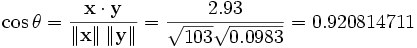Note that the above data were deliberately chosen to be perfectly correlated: y = 0.10 + 0.01 x. The Pearson correlation coefficient must therefore be exactly one. Centering the data (shifting x by E(x) = 3.8 and y by E(y) = 0.138) yields x = (-2.8, -1.8, -0.8, 1.2, 4.2) and y = (-0.028, -0.018, -0.008, 0.012, 0.042), from which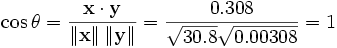as expected.

Principal Component Analysis:

Principal Component Analysis (PCA) involves a mathematical procedure that transforms a number of (possibly) correlated variables into a (smaller) number of uncorrelated variables called principal components. The first principal component accounts for as much of the variability in the data as possible, and each succeeding component accounts for as much of the remaining variability as possible. Each principal component is a linear combinations of all the variables with different coefficients.

### Objectives of principal component analysis

•  To discover or to reduce the dimensionality of the data set.
•  To identify new meaningful underlying variables.

Probability Plot: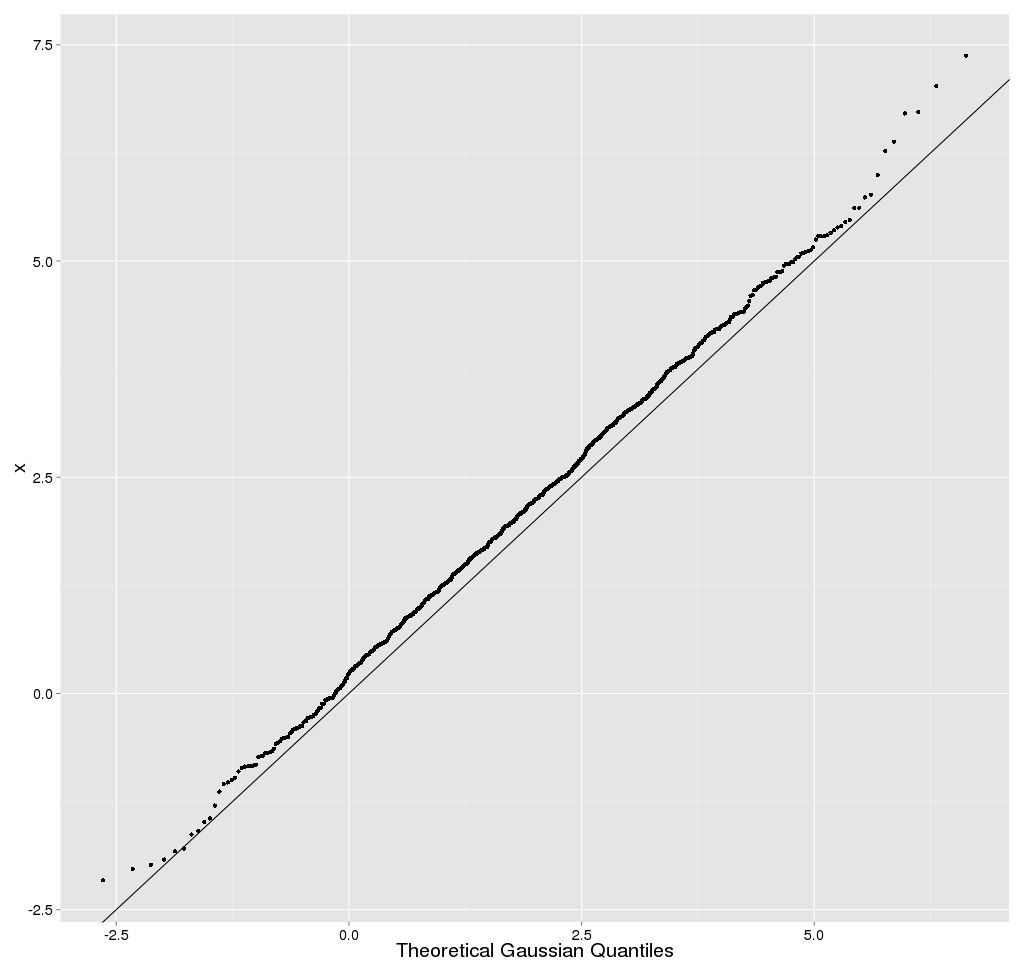Normal Test Plots (also called Normal Probability Plots or Normal Quartile Plots) are used to investigate whether process data exhibit the standard normal "bell curve" or Gaussian distribution.

First, the x-axis is transformed so that a cumulative normal density function will plot in a straight line. Then, using the mean and standard deviation (sigma) which are calculated from the data, the data is transformed to the standard normal values, i.e. where the mean is zero and the standard deviation is one. Then the data points are plotted along the fitted normal line.

The nice thing is that you don't have to understand all the transformations. All you have to do is look at the plotted points, and see how well they fit the normal line. If they fit well, you can safely assume that your process data is normally distributed.

p-value:

Each statistical test has an associated null hypothesis, the p-value is the probability that your sample could have been drawn from the population(s) being tested (or that a more improbable sample could be drawn) given the assumption that the null hypothesis is true. A p-value of .05, for example, indicates that you would have only a 5% chance of drawing the sample being tested if the null hypothesis was actually true.

Null Hypotheses are typically statements of no difference or effect. A p-value close to zero signals that your null hypothesis is false, and typically that a difference is very likely to exist. Large p-values closer to 1 imply that there is no detectable difference for the sample size used. A p-value of 0.05 is a typical threshold .

Top

Quantile-Quantile Plot

(The quantile-quantile (q-q) plot is a graphical technique for determining if two data sets come from populations with a Common distribution.

A q-q plot is a plot of the quantiles of the first data set against the quantiles of the second data set. By a quantile, we mean the fraction (or percent) of points below the given value. That is, the 0.3 (or 30%) quantile is the point at which 30% percent of the data fall below and 70% fall above that value.

A 45-degree reference line is also plotted. If the two sets come from a population with the same distribution, the points should fall approximately along this reference line. The greater the departure from this reference line, the greater the evidence for the conclusion that the two data sets have come from populations with different distributions. )

Top

Sample Generation:

Sample generation is a AstroStat tool that generates random samples of a given size from the specified distribution. These samples are available as CSV files for further use in the application. In addition, to check the distribution of the generated samples, histograms of each are plotted.
To execute this test, input the number of samples and the size of each along with the distribution from which they are to be derived.

Top

Shapiro-Wilks test:

Shapiro-Wilks  test is a formal test of normality offered . This is the standard test for normality. W may be thought of as the correlation between given data and their corresponding normal scores, with W = 1 when the given data are perfectly normal in distribution. When W is significantly smaller than 1, the assumption of normality is not met. That is, a significant W statistic causes the researcher to reject the assumption that the distribution is normal. Shapiro-Wilks W is recommended for small and medium samples up to n = 2000. For larger samples, the Kolmogorov-Smirnov test is recommended.

The Wilks Shapiro test statistic is defined as: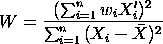where the summation is from 1 to n and n is the number of observations. The array X contains the original data, X' are the ordered data,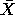is the sample mean of the data, and w'=(w1, w2, ... , wn) or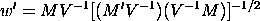M denotes the expected values of standard normal order statistics for a sample of size n and V is the corresponding covariance matrix.

W may be thought of as the squared correlation coefficient between the ordered sample values (X') and the wi. The wi are approximately proportional to the normal scores Mi. W is a measure of the straightness of the normal probability plot, and small values indicate departures from normality.

Top

Linear Regression:

In statistics, linear regression is a method of estimating the conditional expected value of one variable y given the values of some other variable or variables x. The variable of interest, y, is conventionally called the "response variable". The terms "endogenous variable" and "output variable" are also used. The other variables x are called the explanatory variables. The terms "exogenous variables" and "input variables" are also used, along with "predictor variables". The term independent variables is sometimes used, but should be avoided as the variables are not necessarily statistically independent. The explanatory and response variables may be scalars or vectors. Multiple regression includes cases with more than one explanatory variable.

The term explanatory variable suggests that its value can be chosen at will, and the response variable is an effect, i.e., causally dependent on the explanatory variable, as in a stimulus-response model. Although many linear regression models are formulated as models of cause and effect, the direction of causation may just as well go the other way, or indeed there need not be any causal relation at all. For that reason, one may prefer the terms "predictor / response" or "endogenous / exogenous," which do not imply causality.

Regression, in general, is the problem of estimating a conditional expected value.

It is often erroneously thought that the reason the technique is called "linear regression" is that the graph of y = α + βx is a line. But in fact, if the model is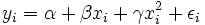(in which case we have put the vector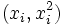in the role formerly played by xi and the vector (β,γ) in the role formerly played by β), then the problem is still one of linear regression, even though the graph is not a straight line.

Linear regression is called "linear" because the relation of the response to the explanatory variables is assumed to be a linear function of some parameters. Regression models which are not a linear function of the parameters are called nonlinear regression models. A neural network is an example of a nonlinear regression model.

Survival:

These techniques were primarily developed in the medical and biological sciences, but they are also widely used in the social and economic sciences, as well as in engineering (reliability and failure time analysis).

To study the effectiveness of a new treatment for a generally terminal disease the major variable of interest is the number of days that the respective patients survive. In principle, one could use the standard parametric and nonparametric statistics for describing the average survival, and for comparing the new treatment with traditional methods. However, at the end of the study there will be patients who survived over the entire study period, in particular among those patients who entered the hospital (and the research project) late in the study; there will be other patients with whom we will have lost contact. Surely, one would not want to exclude all of those patients from the study by declaring them to be missing data (since most of them are "survivors" and, therefore, they reflect on the success of the new treatment method). Those observations, which contain only partial information are called censored observations.

Censored Observations:

In general, censored observations arise whenever the dependent variable of interest represents the time to a terminal event, and the duration of the study is limited in time. Censored observations may occur in a number of different areas of research. For example, in the social sciences we may study the "survival" of marriages, high school drop-out rates (time to drop-out), turnover in organizations, etc. In each case, by the end of the study period, some subjects will still be married, will not have dropped out, or are still working at the same company; thus, those subjects represent censored observations.

In economics we may study the "survival" of new businesses or the "survival" times of products such as automobiles. In quality control research, it is Common practice to study the "survival" of parts under stress (failure time analysis).

Essentially, the methods offered in Survival Analysis address the same research questions as many of the other procedures; however, all methods in Survival Analysis will handle censored data. The life table, survival distribution, and Kaplan-Meier survival function estimation are all descriptive methods for estimating the distribution of survival times from a sample. Several techniques are available for comparing the survival in two or more groups. Finally, Survival Analysis offers several regression models for estimating the relationship of (multiple) continuous variables to survival times.

Based on those numbers and proportions, several additional statistics can be computed:

Number of Cases at Risk. This is the number of cases that entered the respective interval alive, minus half of the number of cases lost or censored in the respective interval.

Proportion Failing. This proportion is computed as the ratio of the number of cases failing in the respective interval, divided by the number of cases at risk in the interval.

Proportion Surviving. This proportion is computed as 1 minus the proportion failing.

Cumulative Proportion Surviving (Survival Function). This is the cumulative proportion of cases surviving up to the respective interval. Since the probabilities of survival are assumed to be independent across the intervals, this probability is computed by multiplying out the probabilities of survival across all previous intervals. The resulting function is also called the survivorship or survival function.

Probability Density. This is the estimated probability of failure in the respective interval, computed per unit of time, that is:

Fi = (Pi-Pi+1) /hi

In this formula, Fi is the respective probability density in the i'th interval, Pi is the estimated cumulative proportion surviving at the beginning of the i'th interval (at the end of interval i-1), Pi+1 is the cumulative proportion surviving at the end of the i'th interval, and hi is the width of the respective interval.

Hazard Rate. The hazard rate (the term was first used by Barlow, 1963) is defined as the probability per time unit that a case that has survived to the beginning of the respective interval will fail in that interval. Specifically, it is computed as the number of failures per time units in the respective interval, divided by the average number of surviving cases at the mid-point of the interval.

Median Survival Time. This is the survival time at which the cumulative survival function is equal to 0.5. Other percentiles (25th and 75th percentile) of the cumulative survival function can be computed accordingly. Note that the 50th percentile (median) for the cumulative survival function is usually not the same as the point in time up to which 50% of the sample survived. (This would only be the case if there were no censored observations prior to this time).

Required Sample Sizes. In order to arrive at reliable estimates of the three major functions (survival, probability density, and hazard) and their standard errors at each time interval the minimum recommended sample size is 30.

Top

Testing for mean when variance is known:

t-test : one sample and two sample tests:

One Sample( test for one mean value):

Let X1,X2,...Xn be a random sample drawn from a Normal population with mean µ and sd σ. Student's t test is used to compare the unknown mean of the population (µ) to a known number (µ0). So here the Null hypothesis is  Ho:  µ =µagainst the alternative H1: µ is not equal to µ0.

Test statistic (population standard deviation σ is known):

The formula for the Z-test is

Z = √n( Sample mean- µ0) / σ

Z has a Normal distribution with mean 0 and variance 1.

Test statistic (population standard σ deviation is unknown):

The formula for t test is t = √n( Sample mean- µ0) / s

Where s is the sample standard deviation.

The statistic t follows t distribution with n-1 degrees of freedom, where n is the number of observations.

Decision of the z or t-test: If the p-value associated with the z or t-test is small (usually set at p < 0.05), there is evidence to reject the null hypothesis in favor of the alternative. In other words, there is evidence that the mean is significantly different than the hypothesized value i.e. the test is significant. If the p-value associated with the z or t-test is not small (p > 0.05), there is not enough evidence to reject the null hypothesis, and you conclude that there is evidence that the mean is not different from the hypothesized value i.e. the test is not significant.

Two sample ( test for equality of two means )

Suppose we have two independent samples

The unpaired t method tests the null hypothesis that the population means related to two independent, random samples from two independent approximately normal distributions are equal against the alternative that they are unequal (as in the one sample case).

Assuming equal variances, the test statistic is calculated as:where x bar 1 and x bar 2 are the sample means, s² is the pooled sample variance, n1 and n2 are the sample sizes and t follows  Student t distribution with n1 + n2 - 2 degrees of freedom.

Paired Sample (from Bivariate Normal Distribution):

The paired t test provides an hypothesis test of the difference between population means for a pair of random samples whose differences are approximately normally distributed.

The test statistic is calculated as:where d bar is the mean difference, s² is the sample variance, n is the sample size and t follows a paired t distribution  with n-1 degrees of freedom.

The decision can be taken exactly in a similar way as in the one sample situation.

Top

Weighted Mean:

The weighted mean is a mean where there is some variation in the relative contribution of individual data values to the mean. Each data value (Xi) has a weight assigned to it (Wi). Data values with larger weights contribute more to the weighted mean and data values with smaller weights contribute less to the weighted mean. The formula is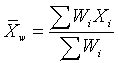There are several reasons why you might want to use a weighted mean.

1. Each individual data value might actually represent a value that is used by multiple people in your sample. The weight, then, is the number of people associated with that particular value.
2. Your sample might deliberately over represent or under represent certain segments of the population. To restore balance, you would place less weight on the over represented segments of the population and greater weight on the under represented segments of the population.
3. Some values in your data sample might be known to be more variable (less precise) than other values. You would place greater weight on those data values known to have greater precision

Wilcoxon rank-sum test:

The Wilcoxon Rank Sum test can be used to test the null hypothesis that two populations X and Y have the same continuous distribution. We assume that we have independent random samples x1, x2, . . ., xm and y1, y2, . . ., yn, of sizes m and n respectively, from each population. We then merge the data and rank of each measurement from lowest to highest. All sequences of ties are assigned an average rank.

The Wilcoxon test statistic W is the sum of the ranks from population X. Assuming that the two populations have the same continuous distribution (and no ties occur), then W has a mean and standard deviation given by

µ = m (m + n + 1) / 2

and

s = √[ m n (N + 1) / 12 ],

where N = m + n.

We test the null hypothesis Ho: No difference in distributions. A one-sided alternative is Ha: first population yields lower measurements. We use this alternative if we expect or see that W is unusually lower than its expected value µ . In this case, the p-value is given by a normal approximation. We let N ~ N( µ , s ) and compute the left-tail P(N <=W) (using continuity correction if W is an integer).

If we expect or see that W is much higher than its expected value, then we should use the alternative Ha: first population yields higher measurements. In this case, the p-value is given by the right-tail P(N >= W), again using continuity correction if needed. If the two sums of ranks from each population are close, then we could use a two-sided alternative Ha: there is a difference in distributions. In this case, the p-value is given by twice the smallest tail value (2*P(N <=W) if W < µ , or 2*P(N >=W) if W > µ ).

We note that if there are ties, then the validity of this test is questionable.

XY Plot:

XY plots(Scatter plots) are similar to line graphs in that they use horizontal and vertical axes to plot data points. However, they have a very specific purpose. Scatter plots show how much one variable is affected by another. The relationship between two variables is called their correlation .

Scatter plots usually consist of a large body of data. The closer the data points come when plotted to making a straight line, the higher the correlation between the two variables, or the stronger the relationship.

If the data points make a straight line going from the origin out to high x- and y-values, then the variables are said to have a positive correlation . If the line goes from a high-value on the y-axis down to a high-value on the x-axis, the variables have a negative correlation .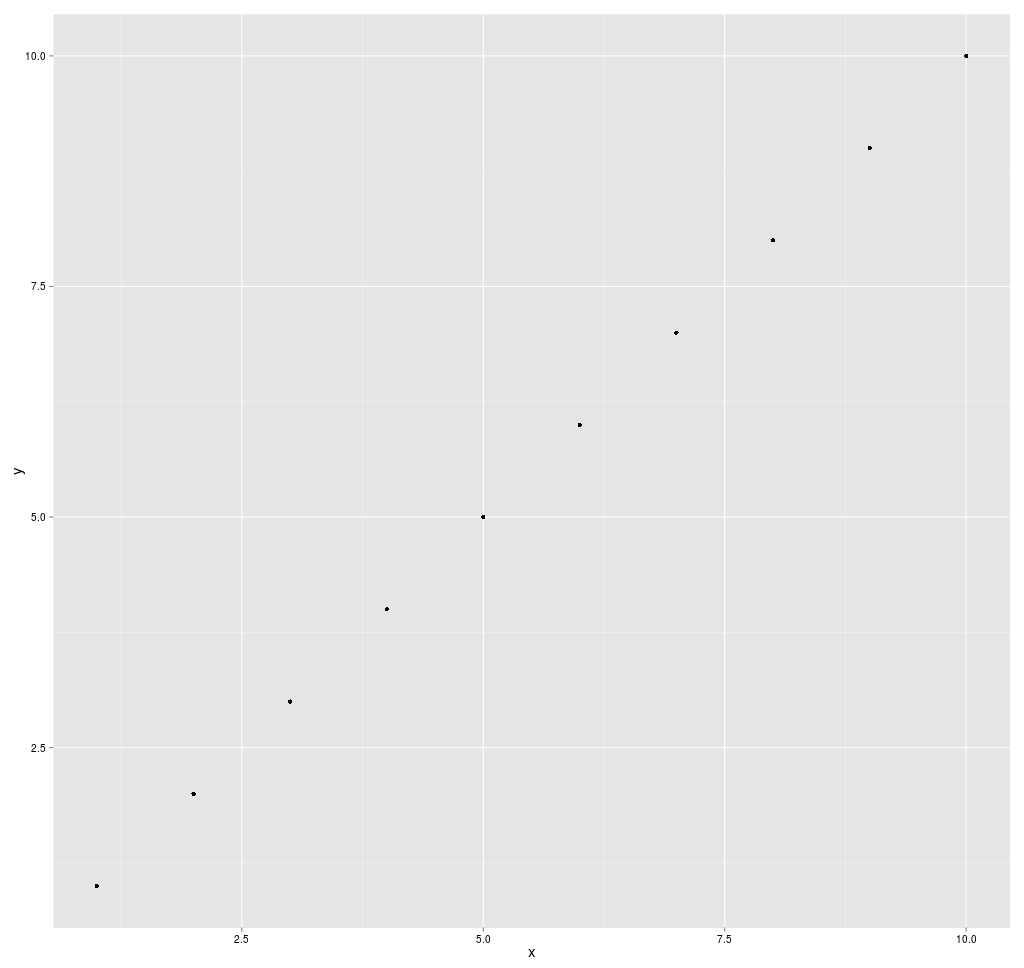A perfect positive correlation is given the value of 1. A perfect negative correlation is given the value of -1. If there is absolutely no correlation present the value given is 0. The closer the number is to 1 or -1, the stronger the correlation, or the stronger the relationship between the variables. The closer the number is to 0, the weaker the correlation. So something that seems to kind of correlate in a positive direction might have a value of 0.67, whereas something with an extremely weak negative correlation might have the value -.21.